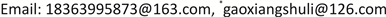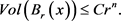﻿ Ricci孤立子的刚性及体积增长 Rigidity and Volume Growth of Ricci Solitons

Pure Mathematics
Vol. 09  No. 01 ( 2019 ), Article ID: 28320 , 9 pages
10.12677/PM.2019.91002

Rigidity and Volume Growth of Ricci Solitons

Jinnan Li, Xiang Gao*

School of Mathematical Sciences, Ocean University of China, Qingdao ShandongReceived: Dec. 5th, 2018; accepted: Dec. 22nd, 2018; published: Dec. 29th, 2018ABSTRACT

After the concept of Ricci flow was proposed by Hamilton in the 1980s and applied to solve the Poincaré conjecture, the classification and geometry of the self-similar solution of Ricci flow (i.e., Ricci soliton) developed rapidly. A gradient soliton is said to be rigid if it is isometric to a quotient of $N×{R}^{k}$ , where $N$ is an Einstein manifold. Volume growth of geodesic balls is an important geometric property for studying manifolds and Ricci soliton and the volume growth rate is an important geometric invariant of manifolds. In this paper, we will systematically summarize the basic development of Ricci solitons, the rigidity and volume growth results of Ricci solitons, and give the conclusion that the complete non-compact gradient Ricci solitons have linear or Euclidean volume growth.

Keywords:Ricci Soliton, Rigidity, Volume GrowthRicci孤立子的刚性及体积增长20世纪80年代Hamilton提出Ricci流的概念并用于解决Poincaré猜想后，Ricci流的自相似解(即Ricci孤立子)的分类及几何结构的研究得到迅速发展。梯度Ricci孤立子为刚性的若它等距于 $N×{R}^{k}$ 的一个有限商空间，其中 $N$ 为爱因斯坦流形。测地球的体积增长是研究流形及Ricci孤立子重要的几何性质，体积增长率也是重要的几何不变量。本文将系统阐述Ricci孤立子的基本发展、Ricci孤立子的刚性及体积增长结果，给出完备非紧致梯度Ricci孤立子线性或欧氏体积增长的结论。Copyright © 2019 by authors and Hans Publishers Inc.1. 引言

1.1. Ricci孤立子介绍

${R}_{ij}+\frac{1}{2}\left({\nabla }_{i}{V}_{j}+{\nabla }_{j}{V}_{i}\right)=\rho {g}_{ij},$

$\rho$ 为常数。此外，若 $V$ 为一个梯度向量场，使得光滑函数 $f$ 满足

${R}_{ij}+{\nabla }_{i}{\nabla }_{j}f=\rho {g}_{ij}.$

$Ric+{\nabla }^{2}f=\rho g$$\rho =0$ 时称为稳定Ricci孤立子， $\rho >0$ 为收缩Ricci孤立子， $\rho <0$ 时称为扩张Ricci孤立子。

1.2. 典型的Ricci孤立子

1) $\left({R}^{n},{g}_{0},\frac{{|x|}^{2}}{4}\right)$ 为带有势函数 $f=\frac{{|x|}^{2}}{4}$ 的梯度收缩孤立子，且 $Ric+{\nabla }^{2}f=\frac{1}{2}{g}_{0}$

2) $\left({R}^{n},{g}_{0},-\frac{{|x|}^{2}}{4}\right)$ 为势函数是 $f=-\frac{{|x|}^{2}}{4}$ 的梯度扩张孤立子，且 $Ric+{\nabla }^{2}f=-\frac{1}{2}{g}_{0}$

2. Ricci孤立子的刚性

Hamilton  (2维情形)及Ivey  (3维情形)证明了紧致Ricci孤立子为刚性的。在Ni-Wallach  工作的基础上，Naber证明了任意具有非负曲率算子的4维完备收缩Ricci孤立子是刚性的，著名的Bryant Ricci孤立子表明存在具有正曲率算子的非刚性旋转对称的稳定及扩张梯度Ricci孤立子。此外不难看出，任意维紧致稳定及扩张梯度Ricci孤立子是刚性的。紧致流形其数量曲率为常数时为刚性的，更一般的，Petersen-Wylie  证明了以下定理：

3. 体积增长估计

Hamilton  系统地开发了Ricci流降低维数的研究方法。由Hamilton对2维Ricci流古典解的分类可知任意具有有界曲率的2维完备古典解都不能达到最大体积增长。在此基础上应用降维方法，Perelman  证明了：

$\underset{r\to +\infty }{\mathrm{lim}}\frac{Vo{l}_{t}\left({B}_{t}\left({x}_{0},r\right)\right)}{{r}^{n}}=0.$

$\underset{r\to \infty }{\mathrm{lim}}\frac{Vol\left({B}_{{x}_{0}}\left(r\right)\right)}{{r}^{n}}=0.$

$Vol\left({B}_{{x}_{0}}\left(r\right)\right)\le C{r}^{n}.$

$Vol\left({B}_{{x}_{0}}\left(r\right)\right)\le C{\left(r+1\right)}^{n-\left(2-\eta \right)\delta }.$

$-r\le f\left(y\right)-f\left(x\right)\le -r+C\mathrm{ln}r.$

$Vol\left(B\left(x,r\right)\right)\le C{r}^{n}.$

$Vol\left(B\left(x,r\right)\right)\le C{r}^{n-\delta }.$

${R}_{ij}+{\nabla }_{i}{\nabla }_{j}f=\frac{1}{2}{g}_{ij}$。则存在常数 $k=k\left({\mu }_{0}\right)>0$，使得当 $r\le 1$$R\le \frac{C}{{r}^{2}}$ 时有：

$Vol\left({B}_{r}\right)\ge k{r}^{n}.$

$Vol\left({B}_{{x}_{0}}\left(r\right)\right)\ge C{r}^{n-2\delta }.$

$Vol\left({B}_{{x}_{0}}\left(r\right)\right)\ge C\mathrm{ln}\mathrm{ln}r.$

$Vol\left({B}_{{x}_{0}}\left(r\right)\right)\ge Cr.$

$\frac{Vol\left({B}_{s}\right)}{{s}^{n}}\le C\cdot {e}^{\frac{-1}{a}{s}^{a}}.$

$\underset{s\to \infty }{\mathrm{lim}}\frac{Vol\left({B}_{s}\right)}{{s}^{n}}\ge \eta .$

$Vol\left({B}_{r}\left(x\right)\right)\ge C{r}^{n}.$$c\cdot r\le Vol\left({B}_{{x}_{0}}\left(r\right)\right)\le c{e}^{a\sqrt{r}}.$

Rigidity and Volume Growth of Ricci Solitons[J]. 理论数学, 2019, 09(01): 11-19. https://doi.org/10.12677/PM.2019.91002

1. 1. Hamilton, R.S. (1998) The Ricci Flow on Surfaces. Contemporary Mathematics, 71, 237-261.
https://doi.org/10.1090/conm/071/954419

2. 2. Perelman, G. (2002) The Entropy Formula for the Ricci Flow and It’s Geometric Applications. arXiv: math.DG/0211159

3. 3. Perelman, G. (2003) Ricci Flow with Surgery on Three-Manifolds. arXiv: math.DG/0303109

4. 4. Perelman, G. (2003) Finite Extinction Time for the Solutions to the Ricci Flow on Certain Three-Manifolds. arXiv: math.DG/0307245

5. 5. Hamilton, R.S. (1982) Three Manifolds with Positive Ricci Curvature. Journal of Differential Geom-etry, 17, 255-306.
https://doi.org/10.4310/jdg/1214436922

6. 6. Hamilton, R.S. (1993) Eternal Solutions to the Ricci Flow. Journal of Differential Geometry, 38, 1-11.
https://doi.org/10.4310/jdg/1214454093

7. 7. Chen, B.L. and Zhu, X.P. (2000) Complete Riemannian Manifolds with Point-Wise Pinched Curvature. Inventiones Mathematicae, 140, 423-452.
https://doi.org/10.1007/s002220000061

8. 8. Cao, H.D. (1996) Existence of Gradient Kähler Ricci Solitons. Elliptic and Parabolic Methods in Geometry (Minneapolis, MM, 1994), A K Peters, Wellesley, MA, 1-16.

9. 9. Sesum, N. (2004) Limiting Behaviour of the Ricci Flow. arXiv: 0402194

10. 10. Hamilton, R.S. (1993) The Formation of Singularities in the Ricci Flow. Surveys in Differential Geometry, 2, 7-136.

11. 11. Ivey, T. (1993) Ricci Solitons on Compact Three-Manifolds. Differential Geometry and its Applications, 3, 301-330.
https://doi.org/10.1016/0926-2245(93)90008-O

12. 12. Hamilton, R.S. (1986) Four-Manifolds with Positive Curvature Operator. Journal of Differential Geometry, 24, 153-179.
https://doi.org/10.4310/jdg/1214440433

13. 13. Böhm, C. and Wilking, B. (2008) Manifolds with Positive Curvature Operator Are Space Forms. Annals of Mathematics, 167, 1079-1097.
https://doi.org/10.4007/annals.2008.167.1079

14. 14. Löpez, M.F. and Río, E.G. (2008) A remark on Compact Ricci Solitons. Mathematische Annalen, 340, 893-896.
https://doi.org/10.1007/s00208-007-0173-4

15. 15. Cao, H.D., Chen, B.L. and Zhu, X.P. (2008) Recent Developments on Ham-ilton’s Ricci Flow. Geometric Flows, Surveys in Differential Geometry, 47-112.

16. 16. Petersen, P. and Wang, W. (2010) On the Classification of Gradient Ricci Solitons. Geometry & Topology, 14, 2277-2300.
https://doi.org/10.2140/gt.2010.14.2277

17. 17. Brendle, S. (2013) Rotational Symmetry of Self-Similar Solutions to the Ricci Flow. Inventiones Mathematicae, 194, 731-764.

18. 18. Brendle, S. (2012) Rotational Symmetry of Ricci Solutions in Higher Dimensions. arXiv: 1203.0270v2

19. 19. Chow, B., Lu, P. and Ni, L. (2006) Hamilton’s Ricci Flow. In: Graduate Studies in Mathematics, Vol. 77, American Mathematical Society Science Press, Providence, RI.

20. 20. Cao, H.D. (1997) Limits of Solutions to the Kähler-Ricci Flow. Journal of Differential Geometry, 45, 257-272.
https://doi.org/10.4310/jdg/1214459797

21. 21. Ni, L. and Wallach, N. (2008) On 4-Dimansional Gradient Shrinking Solitons. International Mathematics Research Notices, 2008, Article ID: rnm152.

22. 22. Petersen, P. and Wylie, W. (2012) Rigidity of Gradient Ricci Solitons. Pacific Journal of Mathematics, 241, 329-345.
https://doi.org/10.2140/pjm.2009.241.329

23. 23. Petersen, P. and Wylie, W. (2009) On Gradient Ricci Solitons with Symmetry. Proceedings of the American Mathematical Society, 137, 2085-2092.
https://doi.org/10.1090/S0002-9939-09-09723-8

24. 24. Lόpez, M.F. and Río, E.G. (2011) Maximum Principles and Gradient Ricci Solitons. Journal of Differential Equations, 251, 73-81.
https://doi.org/10.1016/j.jde.2011.03.020

25. 25. Lόpez, M.F. and Río, E.G. (2011) Rigidity of Shrinking Ricci Solitons. Mathematische Zeitschrift, 269, 461-466.
https://doi.org/10.1007/s00209-010-0745-y

26. 26. Munteanu, O. and Sesum, N. (2013) On Gradient Ricci Solitons. Journal of Geometric Analysis, 23, 539-561.
https://doi.org/10.1007/s12220-011-9252-6

27. 27. Yang, F. and Zhang, L.D. (2017) Rigidity of Gradient Shrinking Ricci Solitons. arXiv:math.DG/1705.09754v1

28. 28. Carrillo, J. and Ni, L. (2009) Sharp Logarithmic Sobolev Inequalities on Gradient Solitons and Applications. Communications in Analysis and Geometry, 17, 721-753.
https://doi.org/10.4310/CAG.2009.v17.n4.a7

29. 29. Cao, H.D. and Zhou, D.T. (2009) On Complete Gradient Shrinking Ricci Solitons. Journal of Differential Geometry, 85, 175-186.
https://doi.org/10.4310/jdg/1287580963

30. 30. Munteanu, O. (2009) The Volume Growth of Complete Gradient Shrinking Ricci Solitons. arXiv: 0904.0798

31. 31. Zhang, S.J. (2011) On a Sharp Volume Estimate for Gradient Ricci Solitons with Scalar Curvature Bounded Below. Mathematics, 27, 871-882.

32. 32. Wei, G.F. and Wu, P. (2013) On Volume Growth of Gradient Steady Ricci Solitons. Pacific Journal of Mathematics, 265, 233-241.
https://doi.org/10.2140/pjm.2013.265.233

33. 33. Cao, H.D. (2011) Geometry of Complete Gradient Shrinking Ricci Solitons. In: Geometry of Complete Gradient Shrinking Ricci Solitons, Geometry and Analysis. No. 1, Adv. Lect. Math. (ALM), Vol. 17, Int. Press, Somerville, MA, 227-246.

34. 34. Munteanu, O. and Wang, J.P. (2012) Analysis of Weighted Laplacian and Applications to Ricci Solitons. Communications in Analysis and Geometry, 20, 55-94.

35. 35. Li, H.Z. and Wei, Y. (2011) Lower Volume Growth Estimates for Self-Shrinkers and Gradient Shrinking Ricci Solitons. arXiv: 1112.0828v1

36. 36. Chen, C.W. (2011) Volume Estimates and the Asymptotic Behavior of Expanding Gradient Ricci Solitons. Annals of Global Analysis and Geometry, 42, 267-277.
https://doi.org/10.1007/s10455-012-9311-7

37. NOTES

*通讯作者。